### Home > MC2 > Chapter 11 > Lesson 11.3.1 > Problem11-83

11-83.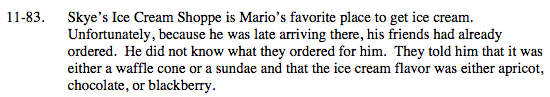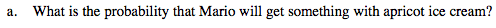Make a tree diagram.

The first choice is between waffle cone or sundae, and each of those could have either apricot, chocolate, or blackberry. How can you put this information into a tree diagram?

How many total combinations are there? How many of those total have apricot ice cream?

$\frac{2}{6} = \frac{1}{3}$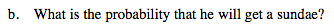How many of the 6 combinations include a sundae?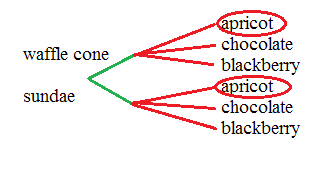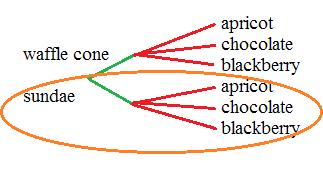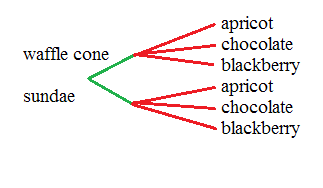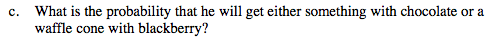See the tree diagram in part (a).

$\frac{1}{3} + \frac{1}{6} = \frac{1}{2}$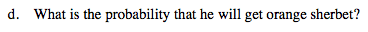How many times does orange sherbet show up in the diagram?